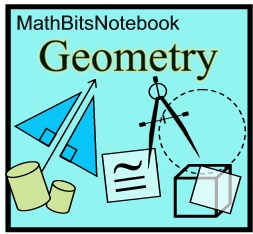Rigid Motions & Congruence MathBitsNotebook.com Terms of Use   Contact Person: Donna Roberts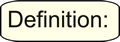Two figures are congruent if and only if there exists one, or more, rigid motions which will map one figure onto the other.

Rigid motions are:
 • reflections • translations • rotationsRigid motions are also called:
 • rigid transformations • isometries • congruence transformations
Rigid motions move figures to a new location without altering their size or shape
(thus maintaining the conditions for the figures to be congruent).

Using Rigid Motion(s) to Determine Congruence: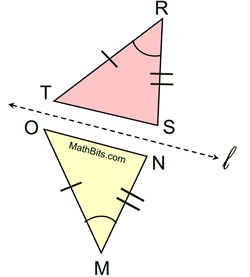Is ΔRST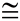ΔMNO? By the definition of congruent, we need to find a rigid motion that will map ΔRST onto ΔMNO. Rigid motion: Reflection A reflection over line l will map ΔRST to coincide with ΔMNO, making ΔRSTΔMNO.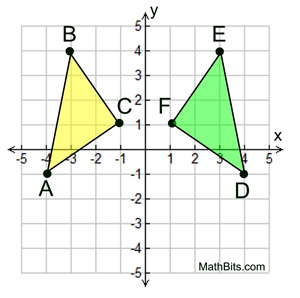Is ΔABCΔDEF? By the definition of congruent, we need to find a rigid motion that will map ΔABC onto ΔDEF. Rigid motion: Reflection A reflection over the y-axis will map ΔABC to coincide with ΔDEF, making ΔABCΔDEF.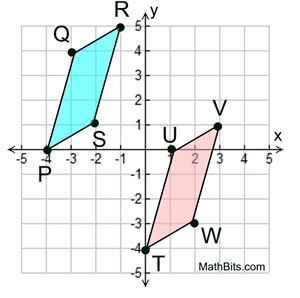Is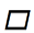PQRSTUVW? We need to find a rigid motion that will map one parallelogram onto the other. Rigid motion: Translation The translation (x, y) → (x + 4, y - 4) will map PQRS onto TUVW, makingPQRSTUVW.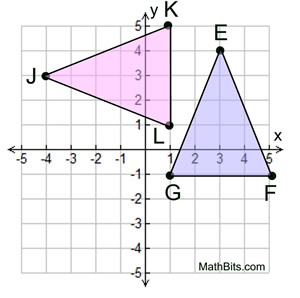Is ΔEFGΔJKL? We need to find a rigid motion that will map one triangle onto the other. Rigid motion: Rotation A rotation of 90º about the origin will map ΔEFG to coincide with ΔJKL, making ΔEFGΔJKL.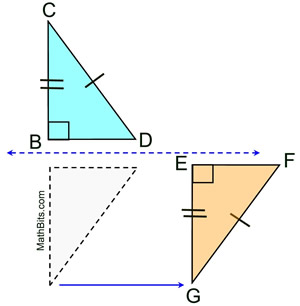Is ΔBCDΔEFG? Sometimes a combination of rigid motions is needed to map one figure onto another. Rigid motions: Reflection and Translation Assuming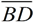and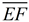are horizontal, reflect ΔBDC over a horizontal line halfway betweenand. Then translate the image horizontally to the right to coincide with ΔEFG making ΔBCDΔEFG.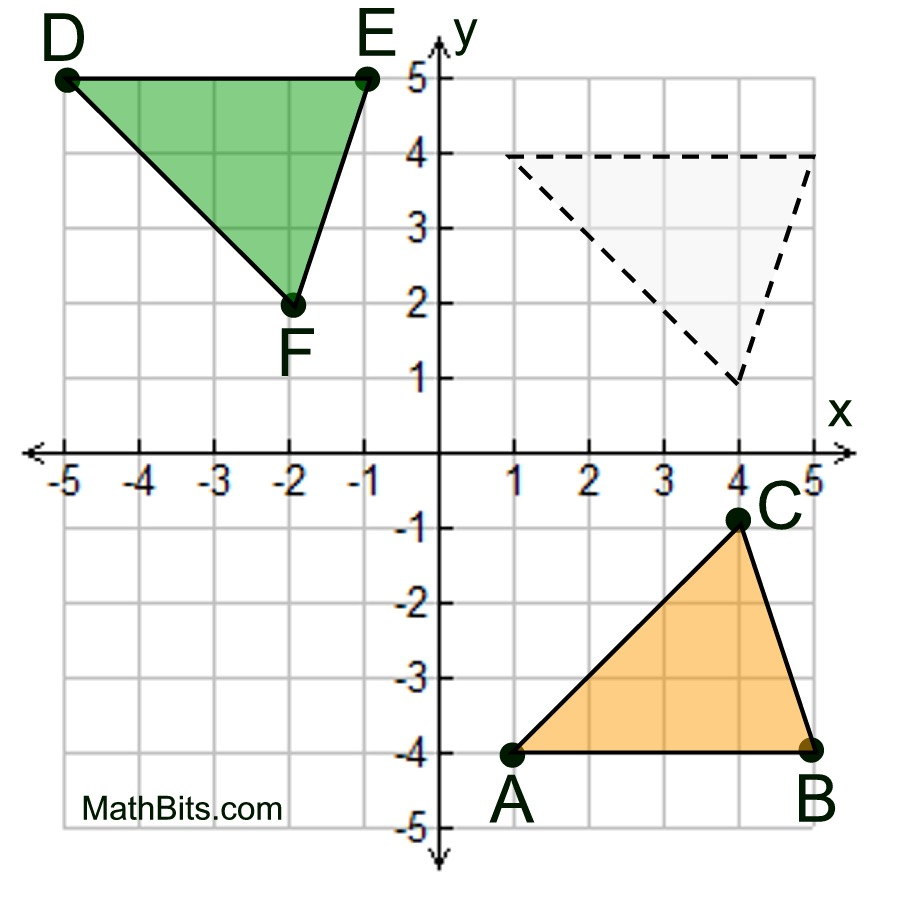Is ΔABCΔDEF? We need to find a combination of rigid motions that will map one triangle onto the other. Rigid motions: Reflection and Translation A reflection in the x-axis, followed by a translation of (x, y) → (x - 6, y + 1), will map ΔABC to coincide with ΔDEF, making ΔABCΔDEF.